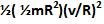## Thursday, December 11, 2008

### Irodov Problem 1.253There are three forces acting on the cylinder as it unrolls downwards, i) the force of gravity mg acting through its center of mass and ii) the tensions T each in the two pieces of string that hold it. Let the acceleration of the cylinder be a. Then we have,

Since the force of gravity passes through the axis of rotation it does not induce any torque on the clinder, however, the tension in each of the strings induces a net torque of 2TR on the cylinder causing it to rotate. Let the angular acceleration of the cylinder be. Since the moment of intertia of the cylinder is, we have,

Since there is no slipping between the string and the cylinder we have,

From, (2) and (3) we can write,

From, (4) and (1) we obtain,

From (5) and (4) we have,

b) At some instant of time if the cylinder is falling at a speed v, its angualr velocity will be v/R.The total energy of the cylinder at any given time is the sum of its translational kinetic energyand its rotational kinetic energy. At any given time the velocity of the cylinder if given by at. Hence we have,

Since all the power is generated by gravity, this must be the power generated by gravity.

#### 1 comment:Anonymous said...

in question 1.254 the cart is going up with accelartion w0 so the force dowmwards should be
(g+w0),how can it be (g-w0)